4564

Chemistry Physical Chemistry Level: Misc Level

The rise in atmospheric carbon dioxide results in higher concentrations of dissolved carbon dioxide in natural waters. Use Henry s law and the following data to calculate solubility of CO2 in water at 25 degrees C when its partial pressure is a) 4.0 k P a b ) 100 k P a

Carbon dioxide Henry s law constant at 25 degrees C =2 .937 K H /k P a m3 /mol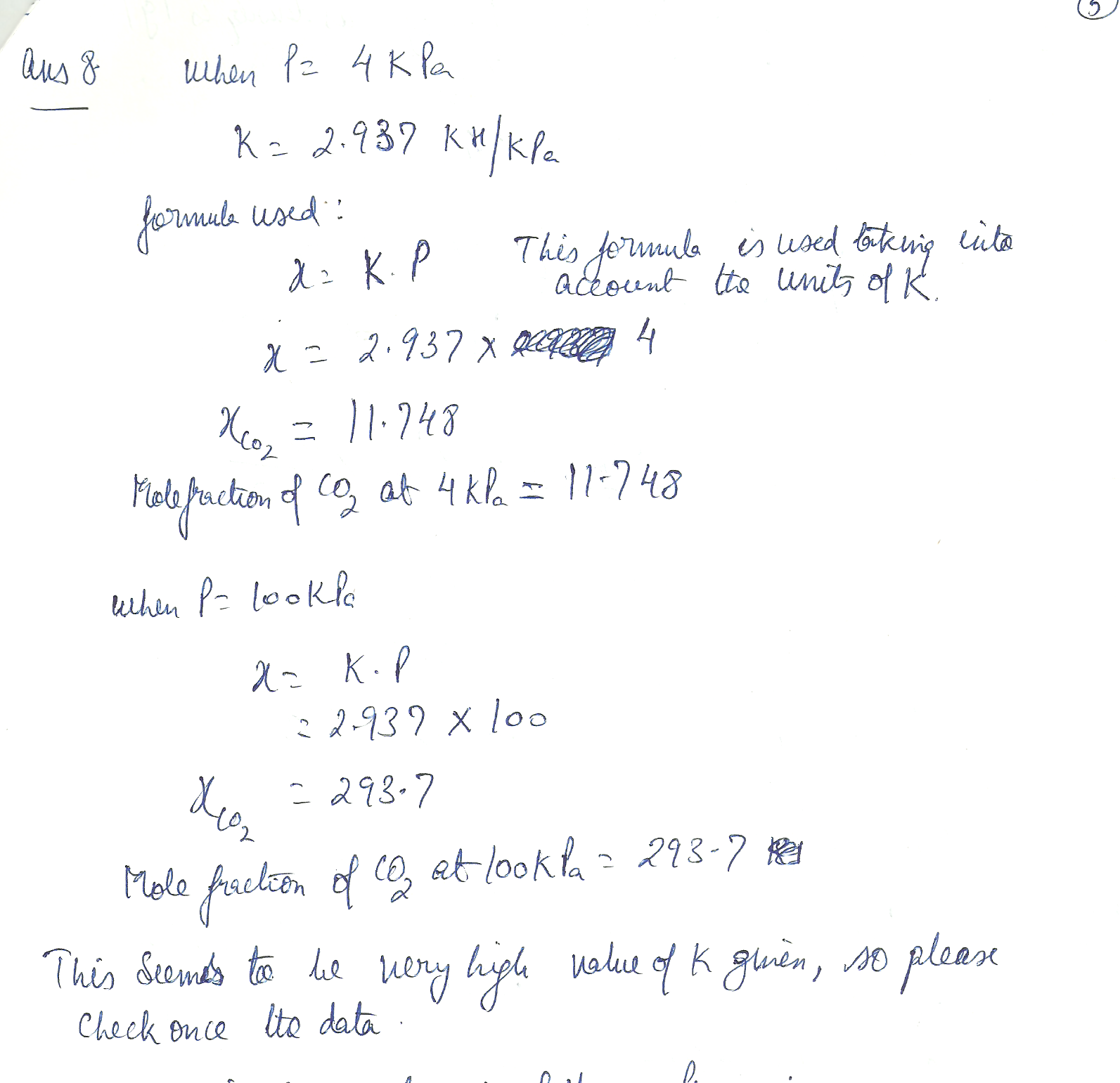4563

Chemistry Physical Chemistry Level: Misc Level

Calculate the concentration of carbon dioxide in fat given that Henry s law constant is

8.6 x 10 to the 4 th Torr and the partial pressure of carbon dioxide is 55 k Pa.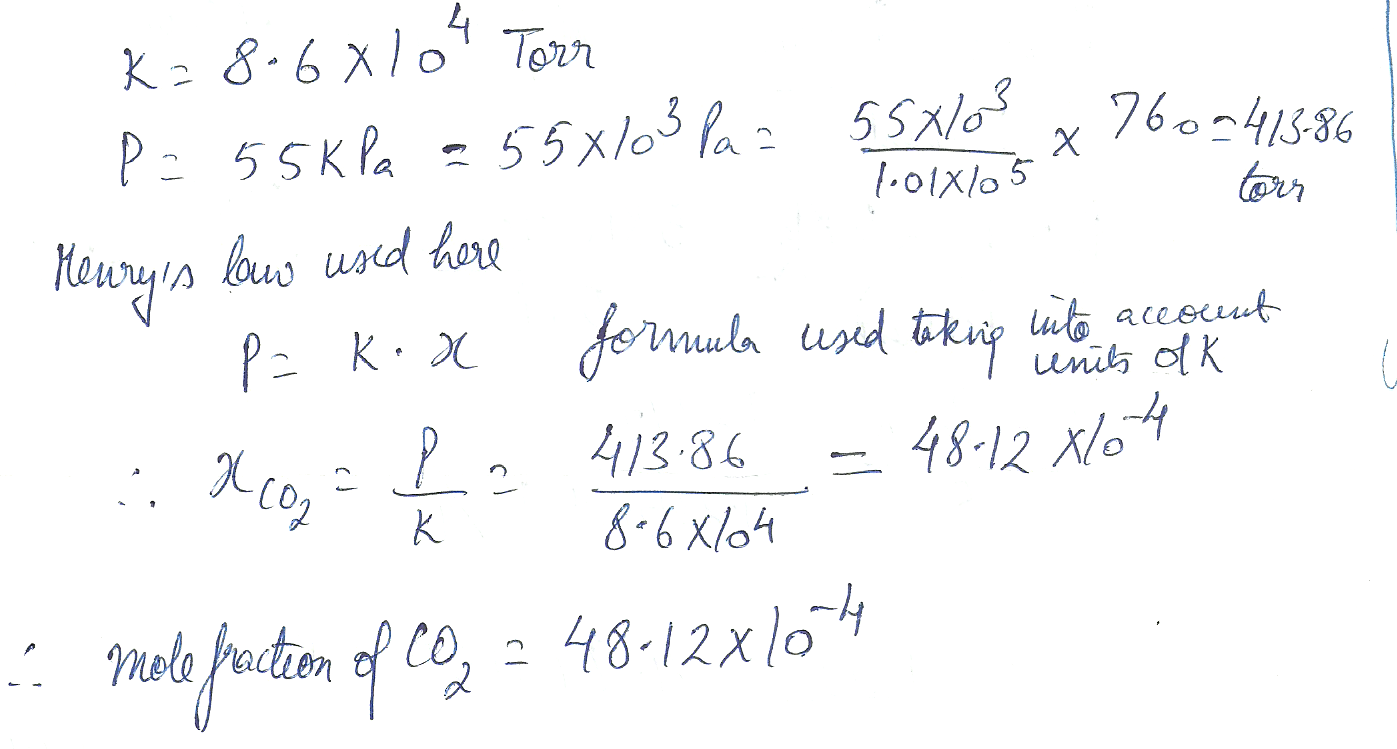4562

Chemistry Physical Chemistry Level: Misc Level

At 300 K, the vapour pressure of dilute solution of H C I in liquid GeCI4 are as follows:

x (HCI ) 0.005 0.012 0.019

p /k Pa 32.0 76.9 121.8

Show that the solution obeys Henry s law in this range of mole fractions and calculate Henry s law constant at 300 K.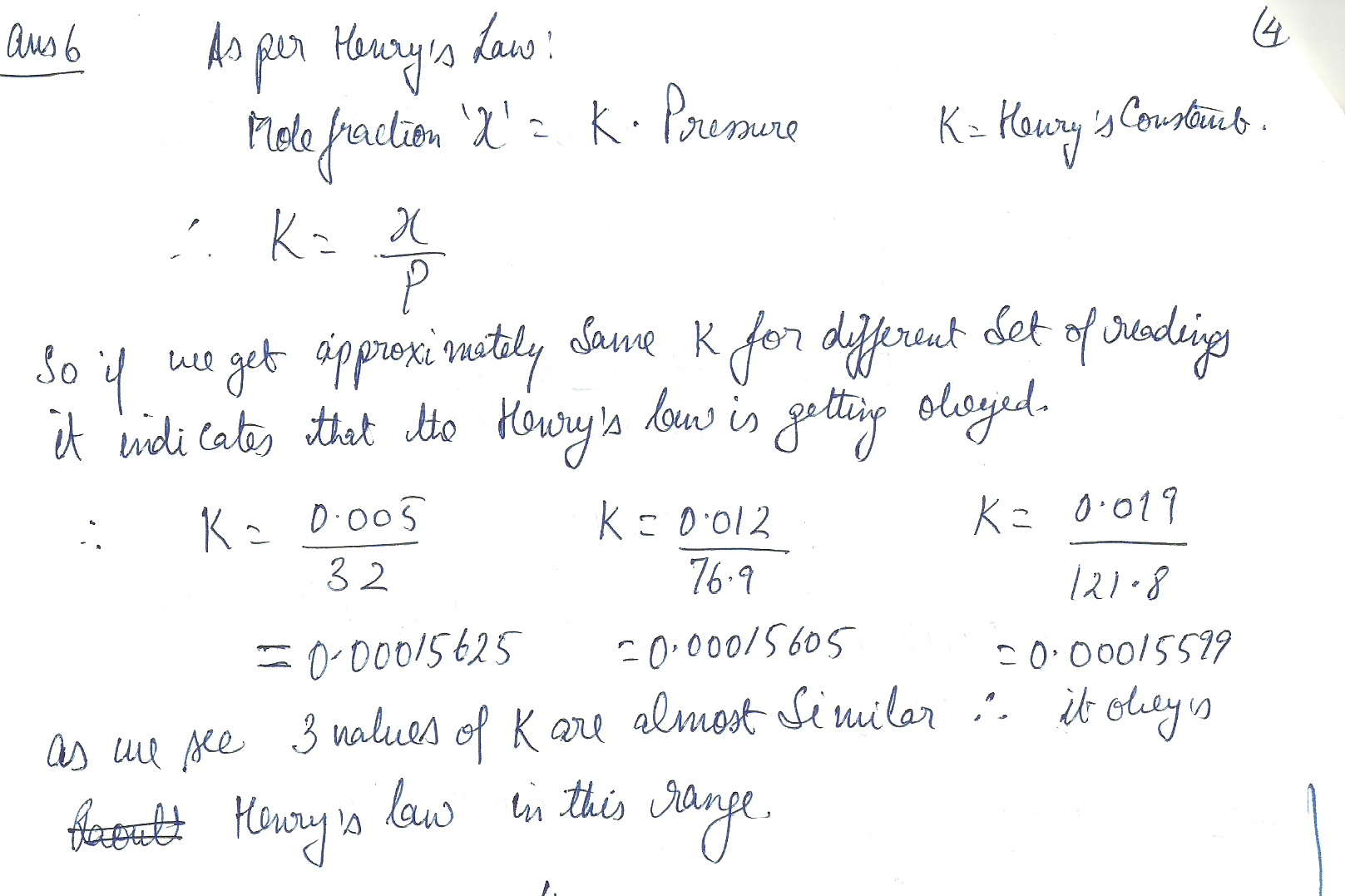4560

Chemistry Physical Chemistry Level: Misc Level

What mass of glucose should you use to prepare 0.112 molal C 6 H 12 O 6 (aq )?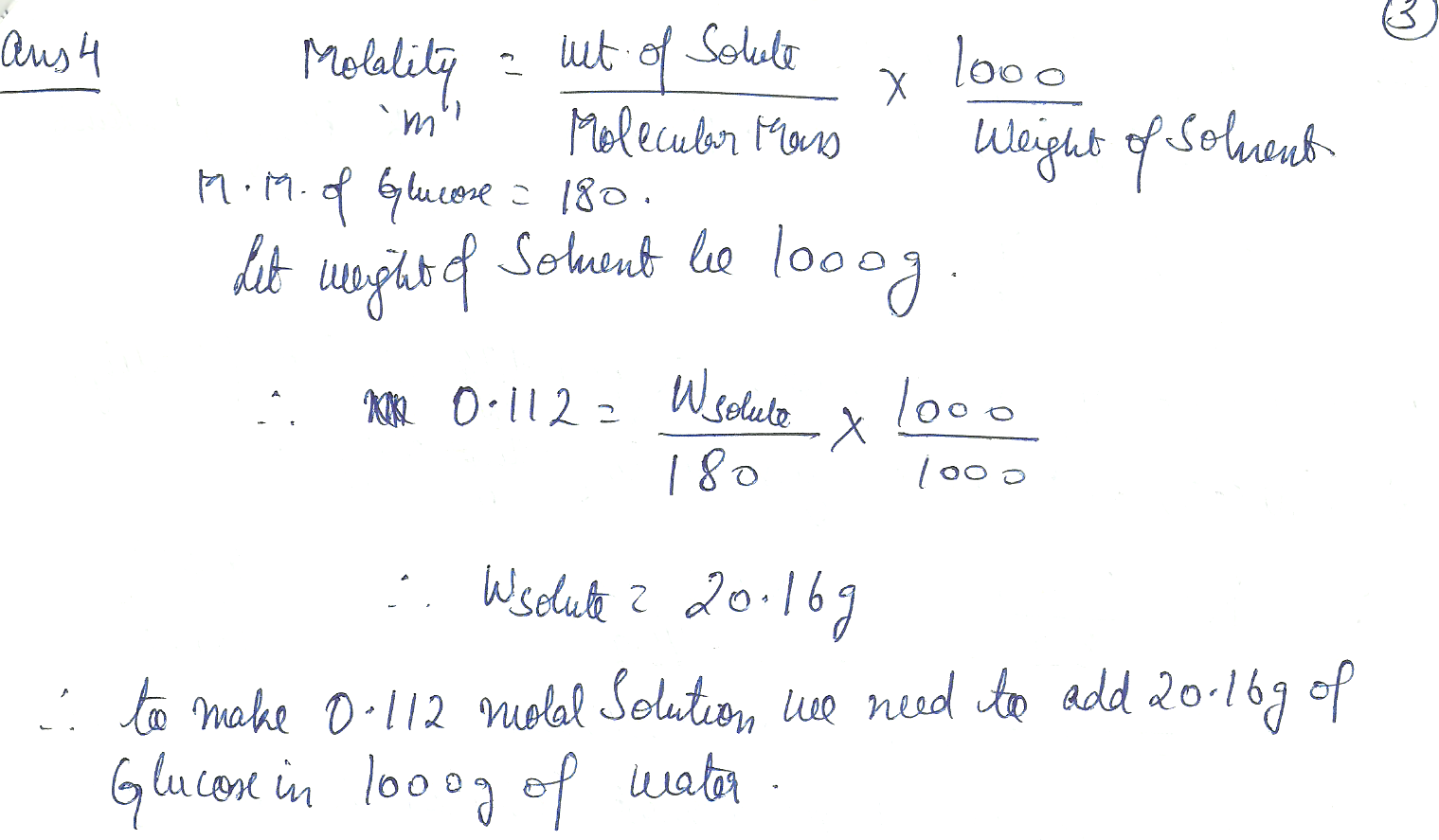4559

Chemistry Physical Chemistry Level: Misc Level

The partial molar volumes of propanone and trichloromethane in a mixture in which the mole fraction of C H C I 3 is 0.4693 are 74.166 cc /mol and 80.235 cc /mol respectively. What is the volume of a solution of total mass 1.000 kg?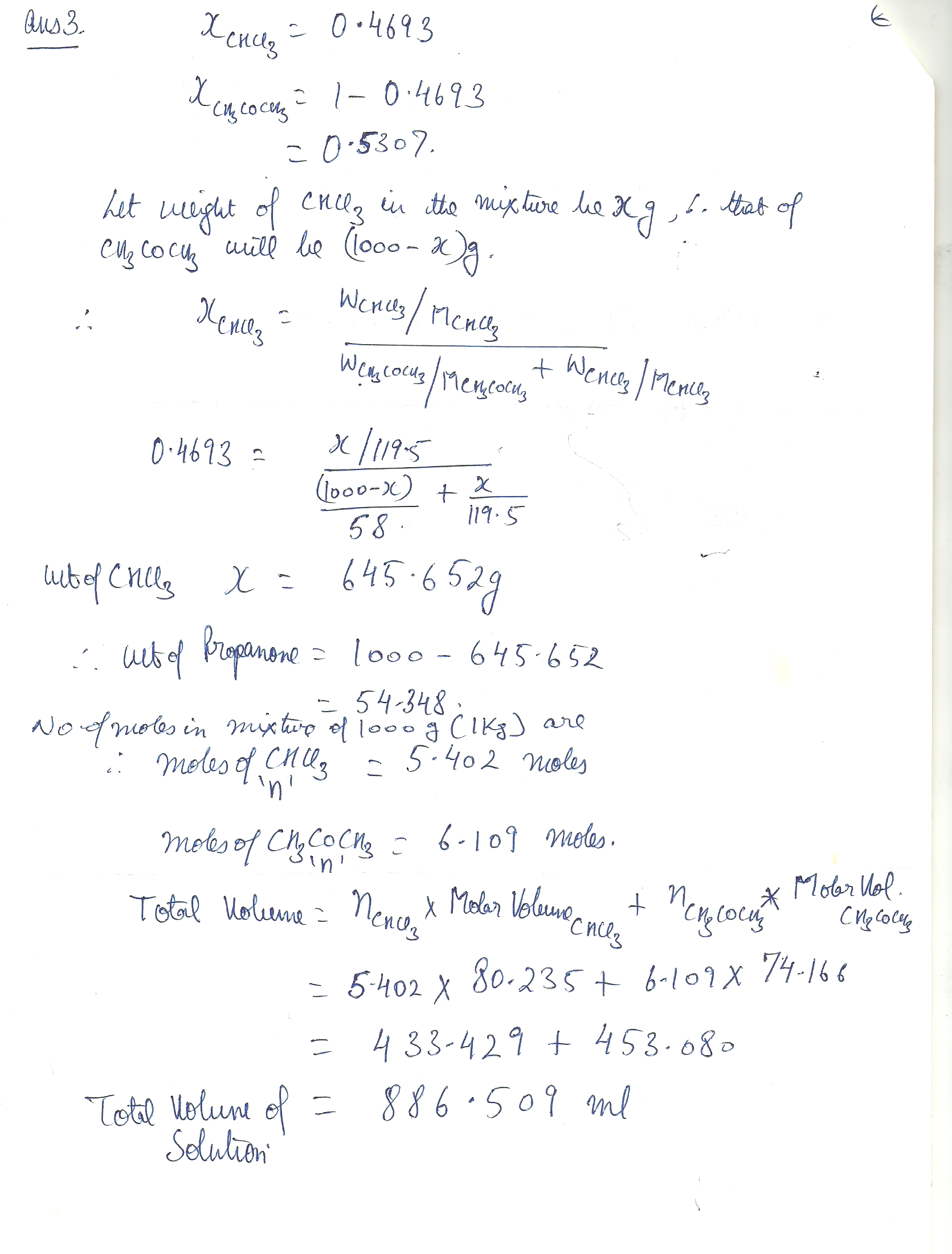4558

Chemistry Physical Chemistry Level: Misc Level

A mixture was prepared consisting of 50.0 g of 1 -propanol and 50.o g of 2 - propanol What are the mole fractions of the two alcohols ?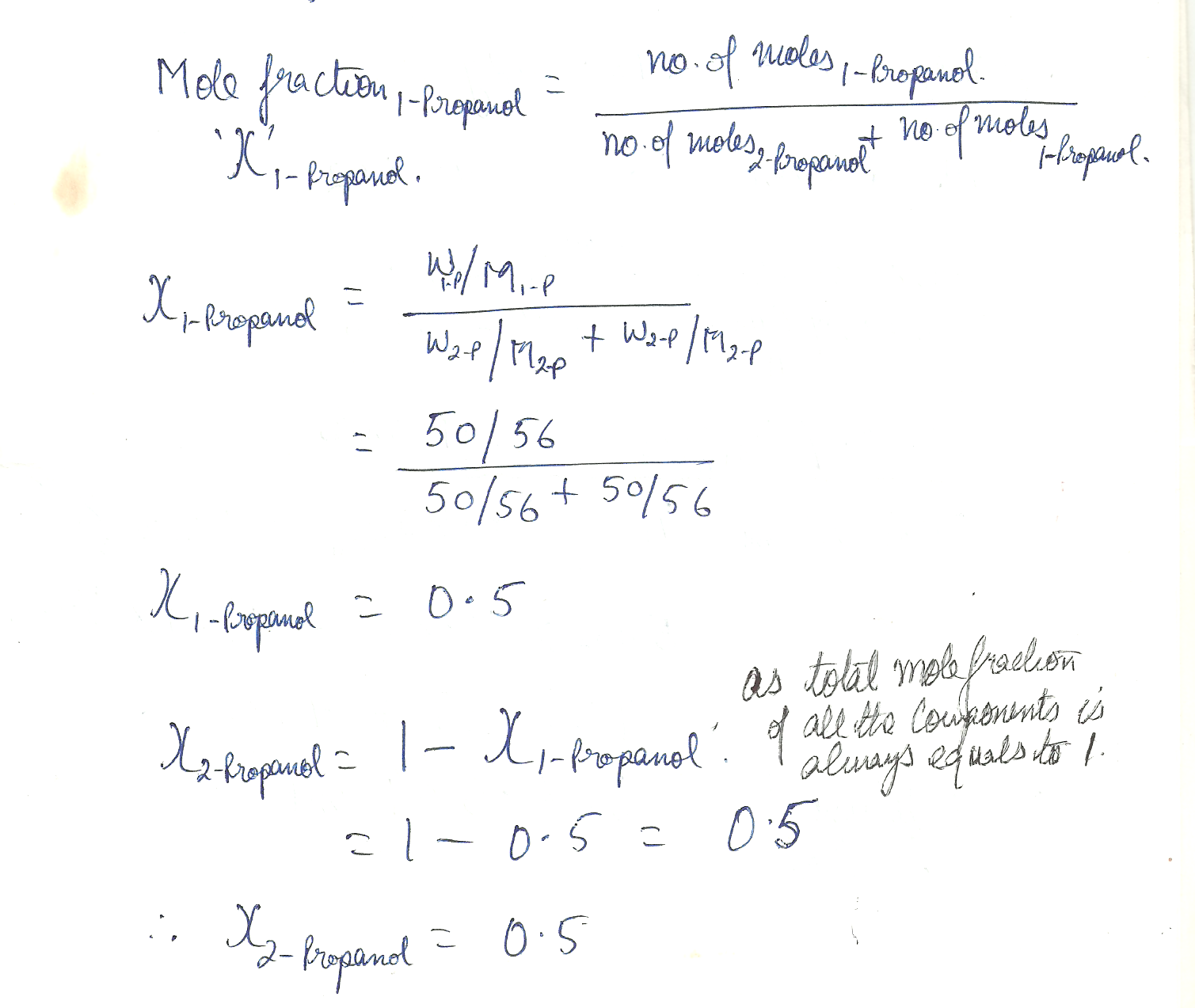Displaying 16-21 of 21 results.